Model Related Instabilities in High Dimensional Linear Models | OMICS International
Journal of Biometrics & Biostatistics

Like us on:

# Model Related Instabilities in High Dimensional Linear Models

Brimacombe M* and Bimali M

Department of Biostatistics, University of Kansas Medical Center, USA

Corresponding Author:
Brimacombe M
Department of Biostatistics
University of Kansas Medical Center
1010 North Kansas, Wichita
KS, 67214, USA
Tel: (913) 588-4785
E-mail: [email protected]

Received Date: April 18, 2016; Accepted Date: May 26, 2016; Published Date: June 05, 2016

Citation: Brimacombe M, Bimali M (2016) Model Related Instabilities in High Dimensional Linear Models. J Biom Biostat 7:308. doi:10.4172/2155-6180.1000308

Copyright: © 2016 Brimacombe M, et al. This is an open-access article distributed under the terms of the Creative Commons Attribution License, which permits unrestricted use, distribution, and reproduction in any medium, provided the original author and source are credited.

Visit for more related articles at Journal of Biometrics & Biostatistics

#### Abstract

The use of high dimensional linear models is common in large database settings. The linearity of such models is often assumed. In sparse settings with the number of subjects (n) less than the number of variables (p) standard algorithms include the lars-LASSO approach which often provides stable convergence. In some cases the underlying data may be more appropriately modeled with a nonlinear model. The use of a linear model in such cases creates model mis-specification and instability for lars-LASSO based approaches. This is studied by using simulations with various relative sample sizes, correlation structures and error distributions.

#### Keywords

High dimensional models; Linear models; Model misspecification; Nonlinear models

#### Introduction

The onset of large databases in many sciences and the need to organize data into information has lead to heightened interest in the analytic and algorithmic methods that guide the analysis of these databases. This has arisen organically in fields such as genomics, imaging, health outcomes, epidemiology and clinical science . Developing stable and interpretable approaches to the organization, analysis and modeling of such databases is a challenge, especially as they often are not subject to formal design standards during data collection.

A very large number of variables and relatively few subjects (large p and small n problems) are typical of such data. Often there is limited theoretical modeling and much of the research is empirically driven, falling under the data science or analytics label . Standard methods of statistical analysis often do not hold up well in such settings . Multiple comparison issues arise requiring careful interpretation and measures of statistical accuracy and significance often lose their meaning.

Correlation and nonlinear relationships may cause difficulty even in the application of simple linear models. The presence of correlated data structures may increase model sensitivity to outliers and anomalies in the data, creating instabilities in the predictive model and affecting identifiability . The mis-specified use of linear models when the data reflect nonlinear patterns will also create bias and other difficulties .

For model-data settings with p>n new techniques and modified models have been developed to deal with such restricted or sparse situations including least angle regression (LARS) and the application of least absolute shrinkage and selection operator (LASSO) . These have been shown to be stable in basic settings where linear models are appropriate. Approaches which extend older statistical techniques include restricted least squares, ridge regression, forward stagewise variable selection and principal components . While application of new "large data" settings are growing , Data Science or BigData approaches to identifying patterns in these large sets of collected data often reflect a mix of algorithmic methods drawn from engineering, computer science and mathematics .

Stability in the linear modeling of large databases is a necessity as modelling in P>n settings requires a restriction of some sort to provide an identifiable model. This is usually a sparsity restriction and we have as a typical model;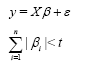for a chosen t. The sparsity restriction imposes onto the linear model context the assumption that only a few of the parameter values will differ from zero. While a variety of different weightings can be used in developing a sparsity restriction, the basic idea of formally limiting the number of variables to be considered remains the same. Typically a forward stage-wise approach is used to fit these models , working within the LASSO assumption that only a few key variables are actually relevant.

In terms of correlations and associations among the variables, if P>n and P is large, work in Hall et al.  and Ahn et al.  examined the P>n situation generally and showed that for large P the data vectors in such a restricted setting cluster at the vertices of an n dimensional simplex. Further these n directions lie approximately perpendicular to each other in forming the simplex structure. This implies that as P→∞ any randomness in the dataset is generated by random rotations of the n vertices of the simplex. The eigenvalues for example from a principal components based analysis of the data converge to equality, limiting the usefulness of clustering methods.

Note that in general the principles of least squares based model fitting provide an approach to obtaining optimal estimators and a fitted model. In the case of using a sparsity restriction, this is not so direct in its application and interpretation. Often there is a phase threshold expressed in terms of P and n at or beyond which least squares based convergence will not occur .

Often more applicable but time consuming is the use of algorithmic search techniques . These cycle through all possible value combinations for the elements of the unknown β vector until an optimal choice is found, minimizing the sum of squared errors subject to the sparsity restrictions. As computers become exponentially faster it is worth noting that such conceptually simpler approaches may become more common .

To examine the stability of sparsity related linear models numerical simulation is a useful approach. True parameter values can be defined, data generated from the true model and the convergence of the resulting estimated model to these true values examined. Nonlinearity and misspecification issues that arise in the analysis of large databases using linear models can be studied. In many settings the true underlying relationship may be a simple nonlinear relationship that can easily be missed when using linear methods or associated correlation based methods. The use of residuals in developing related diagnostic measures of convergence may be difficult as the residuals themselves may be biased and correlated , not at all i.i.d in nature.

Note that in some cases simulated databases should reflect the context of the science within which the statistical models are defined. In the context of population oriented genomics a more genetically relevant process should be used to generate datasets. These should reflect in some way the population genetic structures that exist through generations and underlie the analysis of current generations . Here we define the simulation setting more broadly and do not use such restrictions.

In this paper we study the stability of linear models with sparsity, examining the cases n>p, n=p and p>n. The lars-LASSO approach is the most directly applicable and stable approach to fitting these types of restricted linear models, converging quickly, and is used throughout the simulations. The stability of the method is well understood and has a geometric basis . Here we generate datasets to model a variety of specific distribution and correlation structures in the data, different relative levels of p versus n and mis-specification of the model in question, examining the frequency with which the lars-LASSO based linear model correctly chooses the true set of explanatory variables used to generate the response. The usefulness of these approaches in p>n high dimensional settings is then discussed.

#### Model Stability

Model stability in a linear model can be affected by various properties of the model-data combination. There are some standard challenges and modifications. Sensitivity to rescaling and transformations of the response , the presence of heterogeneity , and use of ridge regression to limit effects of collinearity . These are aimed at improving the application and stability of the model-data combination and resulting fitted model. In the fitting of linear models these issues also extend to diagnostic measures for detecting the effects of outliers and anomalies in the data . From a broader algorithmic perspective, the ordinary least squares estimator can be seen as a very simple yet accurate one-step algorithm, guided and justified by calculus and geometric concepts.

In fitting higher dimensional linear models, the use of residuals in developing diagnostic measures of convergence, a common approach in standard linear models, may be inappropriate when p>n as the residuals may themselves be biased and correlated, not at all i.i.d. in nature. A standard diagnostic measure and fitting criterion is the Cp,m diagnostic which is an estimator based on a total mean square error (MSE) criterion;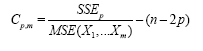where p is the overall number of variables . A property of Cp,m is E[Cp,m]=P, so we can use the value of Cp,m to estimate the number of variables to include in the model. Typically we determine Cp,mfor each value of m choosing the value for p at which the Cp,mvalue stabilizes . This is often viewed graphically. Note that the Cp,m criterion is based on the assumption that the MSE from the full model is an unbiased estimate of σ2.

If the full model happens to contain a large number of parameters that are not significantly different from zero, the MSE will be larger than the estimate of σ2 obtained from a model in which more variables are significant. This is because the variables that are not contributing to decreasing the SSEP are still included in the degrees of freedom when computing MSE. If this is the case, the Cp,m may not be a suitable criterion for determining a useful model . Further if we have a nonlinear component to the data affecting model convergence, then this caveat regarding the application of Cp,m as a model fitting criterion may apply and caution should be exercised.

The LASSO related sparsity restriction that is placed on the set of linear models being considered is given by;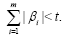This places a restriction on the fitting of the linear models(s) being considered and will alter the set of Cp values to be considered. This is easily incorporated into the lars algorithm .

In the p>n>m setting there is a de facto limitation on the values for Cp,m reflecting the relationship of p to n to m or the set of variables to be considered for at one time. In the case of underlying nonlinearity, the dependence of the measure on the squared length of the orthogonal projection (I – P)y where P = X (X' X )−1 X' and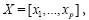gives rise to difficulties as the mis-specified linear model will be biased and as such the mean value of Cp,m may not be useful as a measure of p . Thus the criteria can be easily misapplied when linearity cannot be assumed. If this criteria is an essential aspect of the model fitting approach under assumed linearity, the chosen models may be misleading if the true underlying model is nonlinear. Here we apply the Cp,m criteria throughout for consistency.

#### Results of Numerical Study

The lars-LASSO algorithm was used to fit all models. This is a very stable algorithm with convergence occuring quickly, so simulations were repeated only 30 times in each setting reported here. All models were examined using centered data. Several distributions along with various standard correlation structures (auto-regressive (AR), compound symmetry (CS) and diagonal heterogeneity (DH) with correlations given by 0.8|i–j| and 0.2|i–j|) were examined for various levels of p versus n. Again, the fitting criteria used throughout for comparability is the Cp,m criteria. The overall set of distributions, correlation structures, levels of (n,p) and models considered are summarized in Table 1.

Distributions Correlation Structures p n Model
Normal AR, CS, DH 30, 300 50, 30, 10 Linear, Non-linear
Multivariate-t AR, CS, DH 30, 300 50, 30, 10 Linear, Non-linear
Skew-Normal AR, CS, DH 30, 300 50, 30, 10 Linear, Non-linear

Table 1: Summary of simulation studies.

Using a response y generated from the linear model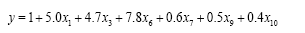in combination with various correlation structures and assumed normal error for all variables x1 to x10, the simulation results given in Table 2 were observed.

CorrelationType, n, p X1 X3 X6 X7 X9 X10 Other
AR, 50, 30 30 30 30 30 30 30 0
AR, 30, 30 30 30 30 30 30 30 0
AR, 10, 30 8 8 24 16 5 4 21
AR, 50,300 26 26 29 29 29 22 7
AR,30, 300 27 29 30 30 28 27 2
AR,10, 300 1 2 3 8 6 3 21

CS, 50, 30 30 30 30 30 30 30 0
CS, 30, 30 30 30 30 30 30 30 0
CS, 10, 30 11 16 20 25 15 8 23
CS, 50,300 28 26 30 28 28 26 7
CS,30, 300 30 30 30 30 29 28 1
CS,10, 300 3 1 8 1 3 2 30

DH1,50,30 30 30 30 30 30 30 0
DH1,30,30 30 30 30 30 30 30 0
DH1,10,30 12 6 17 22 10 6 22
DH1,50,300 27 27 29 29 29 28 2
DH1,30,300 20 19 27 22 15 14 9
DH1,10,300 1 0 7 2 1 0 23

DH2,50, 30 30 30 30 30 30 30 0
DH2,30, 30 30 30 30 30 30 30 0
DH2,10, 30 13 7 19 18 10 6 22
DH2,50,300 27 27 28 29 27 24 6
DH2,30,300 19 18 27 26 16 13 9
DH2,10,300 0 0 10 2 2 0 24

Table 2: Results of Simulations for Various (n,p) Combinations Using A Multivariate Normal with Several Correlation Structures, 30 Replications and Linear Model. Generated response and true model: y=1+5.0x1+4.7x3+7.8x6+0.6x7+0.5x9+0.4x10.

The pattern in this table reveals a large amount of stability in the predictive accuracy of the lars-LASSO approach across various correlation structures. There was some observed instability in models with p >> n and n small and this occured across all correlation structures considered.

Simulations using the same correlation structures but with multivariate-t and skew-normal multivariate error distributions were then conducted and the results shown in Tables 3 and 4. Again there was weakness in variable selection with n small and p >> n across all correlation structures. The more robust error distributions did not alter the general pattern.

Correlation Type, n,p X1 X3 X6 X7 X9 X10 Other
AR, 50, 30 30 30 30 30 30 30 0
AR, 30, 30 30 30 30 30 30 30 0
AR, 10, 30 3 17 24 17 13 6 23
AR, 50,300 29 29 29 27 27 24 8
AR,30, 300 28 28 29 30 24 27 6
AR,10, 300 1 3 11 4 3 1 22

CS, 50, 30 30 30 30 30 30 30 0
CS, 30, 30 30 30 30 30 30 30 0
CS, 10, 30 4 12 12 12 13 4 28
CS, 50,300 30 29 30 29 29 26 3
CS,30, 300 27 25 30 30 22 27 6
CS,10, 300 0 3 6 3 1 0 30

DH1,50,30 30 30 30 30 30 30 0
DH1,30,30 30 30 30 30 30 30 0
DH1,10,30 12 7 14 18 9 4 21
DH1,50,300 27 25 30 28 28 26 7
DH1,30,300 22 16 25 21 11 10 11
DH1,10,300 2 0 7 3 0 0 26

DH2,50, 30 30 30 30 30 30 30 0
DH2,30, 30 30 30 30 30 30 30 0
DH2,10, 30 12 7 17 15 9 4 23
DH2,50,300 26 26 27 29 24 21 8
DH2,30,300 20 16 26 22 13 10 10
DH2,10,300 2 0 7 1 0 0 25

Table 3: Results of Simulations for Various (n,p) Combinations Using A Multivariate-t Distribution with Several Correlation Structures, 30 Replications, and Linear Model: Generated response and true model: y=1+5.0x1+4.7x3+7.8x6+0.6x7 +0.5x9+0.4x10.

Correlation Type, n, p X1 X3 X6 X7 X9 X10 Other
AR, 50, 30 30 30 30 30 30 30 0
AR, 30, 30 30 30 30 30 30 30 0
AR, 10, 30 7 14 23 25 9 6 12
AR, 50,300 29 24 29 28 25 25 10
AR,30, 300 27 27 30 28 28 25 4
AR,10, 300 2 3 18 10 6 0 21

CS, 50, 30 30 30 30 30 30 30 0
CS, 30, 30 30 30 30 30 30 30 0
CS, 10, 30 11 20 26 18 14 5 19
CS, 50,300 28 28 26 29 29 26 7
CS,30, 300 19 20 29 25 23 20 9
CS,10, 300 4 5 8 4 1 0 30

DH1,50,30 30 30 30 30 30 30 0
DH1,30,30 30 30 30 30 30 30 0
DH1,10,30 11 12 17 18 10 11 12
DH1,50,300 29 29 30 29 29 30 2
DH1,30,300 21 19 30 24 18 16 6
DH1,10,300 2 1 6 5 4 3 23

DH2,50, 30 30 30 30 30 30 30 0
DH2,30, 30 30 30 30 30 30 30 0
DH2,10, 30 11 12 15 18 11 11 12
DH2,50,300 29 29 30 29 29 30 3
DH2,30,300 21 19 30 24 16 14 6
DH2,10,300 3 1 8 3 4 3 22

Table 4: Results of Simulations for Various (n,p) Combinations Using A Multivariate Skew Normal Distribution with Several Correlation Structures, 30 Replications, and Linear Model: Generated response and true model: y=1+5.0x1+4.7x3+7.8x6+0.6x7 +0.5x9+0.4x10.

The same basic linear model fitting was then examined from the perspective of total variation explained, with results given in Table 4. For p=5000 and n=100, 250 and 500 using the same set of error distributions and correlation structures, the number of principal components  accounting for 75-80% of variation was determined for all models. Note that in the p>n case the number of principal components is limited by the sample size n and the percent reported here is the number of principal components divided by the number of available eigenvectors.

As shown in Table 5 the percent of non-zero principal components required to explain 75-80% of total variation was in the 45-68% range of non-zero principal components. For all distributions and correlation structures, as n decreased in relation to p, the percent of principal components required to explain the given level of total variation in the data increased. This reflects the geometric arguments mentioned in [8,9] as the principal components here converge towards equality and more of them are required to explain a given level of total variation.

,tbody>
Distribution Covariance
Structure
Number of Principal Components (% Non-zero components)
Normal Autoregressive 68 (68%)
159 (64%)
293 (59%)
Compound Symmetry 44 (44%)
102 (41%)
187 (37%)
Diagonal Heterogeneity 70 (70%)
166 (66%)
312 (62%)

Multivariate-t Autoregressive 50 (50%)
114 (46%)
216 (43%)
Compound Symmetry 29 (29%)
57 (23%)
113 (23%)
Diagonal Heterogeneity 51 (51%)
117 (47%)
227 (45%)

Skew-Normal Autoregressive 65 (65%)
149 (60%)
264 (53%)
Compound Symmetry 60 (60%)
136 (54%)
242 (48%)
Diagonal Heterogeneity 67 (67%)
158 (63%)
289 (58%)

Table 5: Number of Principal Components Accounting for 75-80% of Total Variation for p=5000 and (n=100, 250 and 500).

The compound symmmetry correlation structure leads to slightly lower levels of variation explained. This pattern held for all error distributions with slightly lower levels in the multivariate-t distribution case where outlier generation is more common.

Overall, the results reported in Table 1 through Table 4 indicate the stability of the lars-LASSO stagewise approach in linear models, especially when p ≤ n. For levels of p >> n the predictive aspect of the model is weak across the various types of correlation structures and error distributions considered.

Nonlinearity and model mis-specification

The use of a linear model when underlying nonlinearity exists in the data can lead to misleading results. The curvature of the underlying nonlinear model can be substantial and effect the accuracy of the model . This was examined here using a simulated partially nonlinear regression model of the form;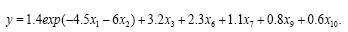Restricting our study to cases of p ≥ n, nonlinearity had a strong effect on the model fitting, even when limited to only a few variables. We deliberately mis-specified the model using a linear model for fitting when the underlying relationship in the data was nonlinear with the Cp,m criteria used for comparative purposes, but may this not be stable for variable selection. Thirty replications were simulated using n=(10,30,50) and p=(30,300) and a similar set of error distributions and correlation structures as in Tables 1-4 with a linear model assumption.

The results for the nonlinear models are given in Tables 6-8.

Distribution Covariance
Structure
n p X1 X2 X3 X6 X7 X9 X10
Normal Autoregressive 10 30 1 0 1 2 2 6 4
10 300 0 0 0 0 0 0 0
30 30 11 9 22 16 10 13 13
30 300 0 0 2 0 2 2 1
50 30 5 8 22 18 11 12 13
50 300 0 0 0 2 3 3 3
Compound Symmetry 10 30 2 2 5 7 2 2 2
10 300 0 0 0 0 0 0 0
30 30 16 5 20 10 10 18 10
30 300 1 0 1 7 0 1 1
50 30 8 5 23 13 6 15 12
50 300 0 0 6 1 10 1 4
Diagonal Heterogeneity 10 30 0 0 5 6 1 5 8
10 300 0 0 0 3 0 0 0
30 30 11 5 15 16 14 11 14
30 300 0 0 1 0 3 7 1
50 30 0 7 15 11 14 20 12
50 300 0 0 2 0 0 2 2

Table 6: Variable Selection Rates for 30 Replications, Normal Error and Various Correlation Structures.

Distribution Covariance
Structure
n p X1 X2 X3 X6 X7 X9 X10
Multivariate-t Autoregressive 10 30 0 0 0 3 3 7 10
10 300 0 0 0 0 0 1 1
30 30 10 9 16 15 17 21 20
30 300 0 0 0 2 0 0 2
50 30 0 0 3 2 3 6 0
50 300 0 0 0 5 6 12 1
Compound Symmetry 10 30 0 0 4 5 0 8 6
10 300 0 0 0 0 0 0 0
30 30 7 8 11 18 17 18 15
30 300 0 0 0 0 1 0 0
50 30 8 4 9 10 21 16 12
50 300 1 0 1 0 6 8 1
Diagonal Heterogeneity 10 30 0 0 5 10 7 10 11
10 300 0 0 0 0 0 1 3
30 30 10 14 14 20 19 21 17
30 300 0 0 2 4 0 3 1
50 30 12 11 21 19 17 10 17
50 300 0 0 0 3 0 1 2

Table 7: Variable Selection Rates for 30 Replications, Multivariate-t Error and Several Correlation Structures.

Distribution Covariance
Structure
n p X1 X2 X3 X6 X7 X9 X10
Skew-Normal Autoregressive 10 30 0 0 1 3 7 3 6
10 300 0 0 0 0 0 0 0
30 30 10 12 15 9 13 13 19
30 300 0 0 0 0 0 1 5
50 30 2 6 2 29 15 22 4
50 300 0 0 0 6 7 1 1
Compound Symmetry 10 30 0 0 5 5 2 10 2
10 300 0 0 2 0 1 0 0
30 30 12 11 13 12 17 9 16
30 300 0 0 0 0 0 3 1
50 30 0 6 5 29 13 9 0
50 300 0 0 0 2 3 3 3
Diagonal Heterogeneity 10 30 0 0 3 1 3 11 2
10 300 0 0 0 0 1 0 0
30 30 11 11 14 12 12 14 18
30 300 0 0 0 1 0 3 1
50 30 1 2 2 28 13 7 0
50 300 0 0 0 6 2 1 0

Table 8: Variable Selection Rates for 30 Replications, Skew-Normal Error and Various Correlation Structures.

While some discussion of error distribution and correlation structure may be useful, we can see that the mis-specification of a linear model when the true model is nonlinear gives poor results. Variable selection is uniformly poor and the rate at which the model picks up variables that have little or no support in the actual simulation is relatively high. The cause of this is the nature of the lars algorithm which uses orthogonal projections of the remaining residual vectors at each stage to find the next predictive variable to which it is highly correlated.

In the linear model setting this algorithm creates a fast stepwise convergence to an optimal answer. However the use of both orthogonal projection and correlation is challenging when nonlinearity is present. Nonlinear patterns are less likely to be detected when correlation is present and orthogonal projections onto linear subspaces are not appropriate by definition when model fitting with nonlinear models . In addition, as noted above the Cp,m criteria is poorly defined and can be misleading.

Mis-specification and sparsity restriction

The issue of mis-specification arises as there is no guarantee that the use of a linear model will necessarily agree with the underlying data structure, as seen above. Problems will arise if the data are actually nonlinear in pattern. This is common in many natural phenomena, especially in relation to growth patterns. When this is the case models that focus on linearity may miss non-linear patterns in the data. The effects of nonlinearity can be difficult to deal with as the least squares or maximum likelihood estimates in fitting models may be correlated and correlation can be very misleading in the presence of noninear patterns. Thus the effects of mis-specification in regard to a small number of variables will impact the fitting of the entire model.

To more formally address the mis-specification issue with sparsity we express the linear model as function of two sets of variables;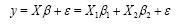where initially n>p. Let us assume that the key significant variables are grouped in the X1 (n × p1) matrix with p1 variables, the X2 (n × p2) matrix has p2 additional variables, where p1 << p2 and p1 + p2=p. The error term ε (n × 1) is assumed to have the distribution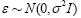. The goal here is to identify the variables in the X1 matrix. Now assume the aspect of interest is a nonlinear model underlying the X1 set of variables. In this setting we wish to assess to what extent the LASSO  or related technique may not detect the set of variables embedded in the nonlinear model. We re-express our initial model as;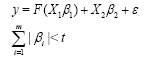where F(X1 β1) is a nonlinear model for the X1 subset of variables and we focus the sparsity restriction on the X1 set of variables, selecting k<p1 non-zero coefficients in the sparsity restriction. Replacing F(X1 β1) with its Taylor expansion about β10 to the second order we obtain;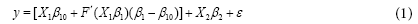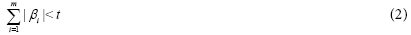If we were to apply a linear model to this setting we would in essence be using a local linear approximation rather than the true model, giving;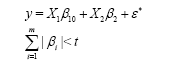where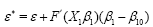and in fitting this, we will both potentially miss the nonlinear aspect of the data and apply an approach which employs a biased error distribution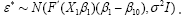In the p>n setting sparseness will not help alleviate the misspecification issue. Indeed the mis-specification effect may be augmented over the restrictions implicit in the use of the sparsity restriction. The Cp,m criteria defined here in this context will not be unbiased  and use of residuals in diagnostic measures may be inappropriate as noted above.

Note that if the underlying model is mis-specified, the sparseness restriction may not actually make sense, as it applies a linear scale to the relative importance of the estimated parameter coefficients. Note further that the centering of the data, a key component in the LASSO-lars algorithm , may actually increase the bias in the model if there is underlying nonlinearity . Indeed the effect of centering in such a mis-specified settings may alter depending on each set of variables considered in the stagewise fitting process.

#### Discussion

The simulation and study of model fitting behavior in high dimensional settings is an interesting use of the computer as a tool of scientific inquiry. Simulation allows for the study of algorithm behavior across a wide set of assumptions. Here the accuracy and stability of the lars-LASSO algorithm in linear models with n>p and p>n to a lesser extent is demonstrated in this manner. The number of replications has been kept fairly small due to the known stabiility and accuray of the algorithm investigated.

Use of mis-specified nonlinear models can lead to a high level of observed instability and model sensitivity. The assumption of linearity in large databases is often a common approach to the initial modeling of the variables measured. If there is a scientific context for the variables in question this should be reviewed to ensure that a linear scaling is appropriate. Note that it may be necessary to rescale some variables before a linear predictive model is appropriate for application.

Standard diagnostic assessments of model robustness and fit must be carefully interpreted in these settings. Numerical studies conducted here show that nonlinearity and p>n are a potential basis of predictive error in high dimensional model settings. Further potential misspecification issues may be present, affecting the accuracy of predictive linear models. The lars-LASSO model can be extended by altering the definition of sparsity, but this does not necessarily imply robustness to nonlinear specification and correlation structure.

#### References

Select your language of interest to view the total content in your interested language

### Article Usage

• Total views: 8672
• [From(publication date):
June-2016 - Dec 08, 2019]
• Breakdown by view type
• HTML page views : 8572Can't read the image? click here to refresh